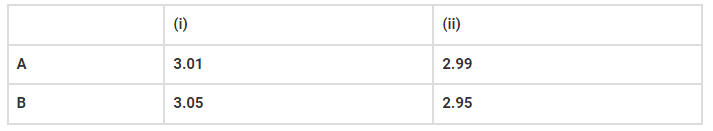# Two students performed the same experimentQuestion:

Two students performed the same experiment separately and each one of

them recorded two readings of mass which are given below. The correct reading

of mass is 3.0 g. Based on given data, mark the correct option out of the

following statements.(i) Results of both the students are neither accurate nor precise.

(ii) Results of student A are both precise and accurate.

(iii) Results of student B are neither precise nor accurate.

(iv) Results of student B are both precise and accurate.

Solution: